﻿ 记忆依赖型偏微分方程数值解研究 On Numerical Solution of the Memory Dependent Partial Differential Equations

Vol.06 No.04(2017), Article ID:21532,7 pages
10.12677/AAM.2017.64074

On Numerical Solution of the Memory Dependent Partial Differential Equations

Wenwen Sun, Jinliang Wang

Research Institute for ESMD Method and Its Applications, College of science, Qingdao University of Technology, Qingdao Shandong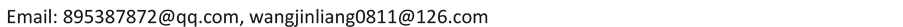Received: Jul. 7th, 2017; accepted: Jul. 24th, 2017; published: Jul. 27th, 2017ABSTRACT

If the rate of change respect to time in the classical string-vibration equation and heat-conduction equation is replaced by the type of memory-dependent derivative, what is the difference for the behavior of the solution? To compare with the ordinary derivative, the memory-dependent type can reflect clearly the dependence of physical process on their past states. To compare with the fractional derivative, the kernel function can be chosen freely and the interval for dependence doesn’t increase with time; so the memory-dependent partial differential equation should have strong expressive force. In this study, the case of kernel function with linear form is considered. Numerical results show that: 1) The characteristics of the solution lie between the string-vibration equation and the heat-conduction equation. It has both fluctuating and decaying properties. The amplitude of it decreases along with the increasing of time-delay and diffusion-coefficient. 2) To compare with the Caputo type of fractional partial differential equation, the fluctuation of the solution is stronger and the decay of amplitude is slower.

Keywords:Memory-Dependent Derivative, Memory-Dependent Partial Differential Equation, Caputo Fractional Derivative, String Vibration Equation, Heat Conduction Equation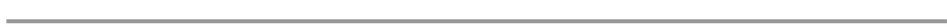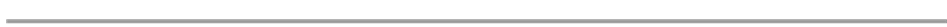1. 引言

2. 记忆依赖型偏微分方程

2.1. 记忆依赖型导数(MD)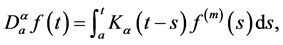(1)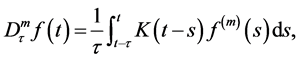(2)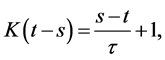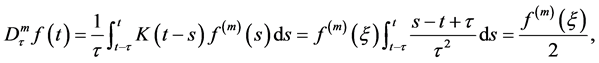(3)

2.2. 记忆依赖型偏微分方程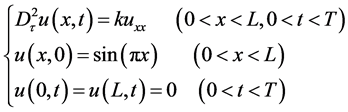(4)3. 与经典的热传导方程及波动方程的比较(5)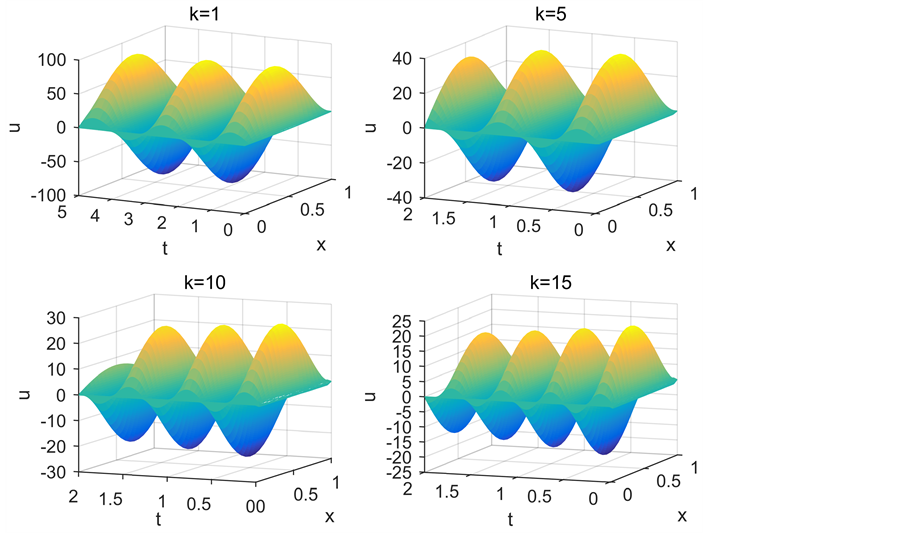Figure 1. The influence of different diffusion coefficients on the solutions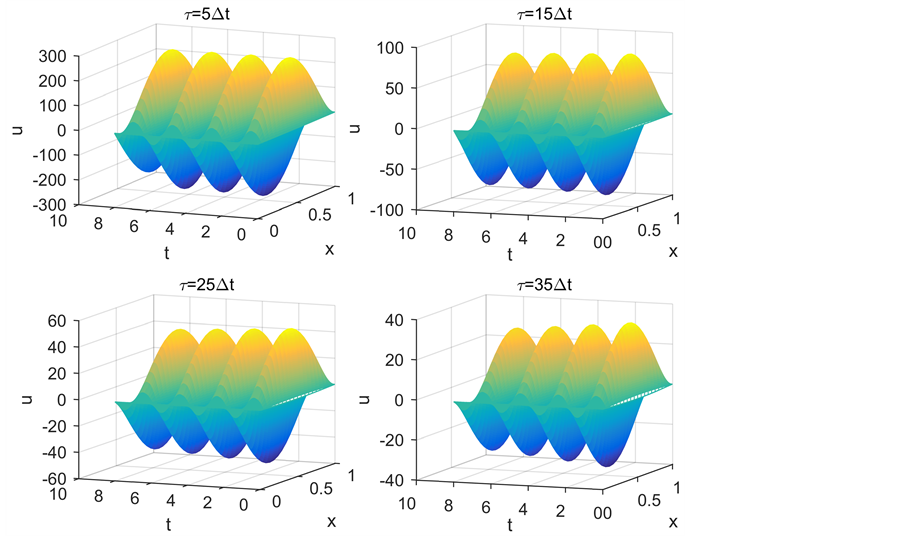Figure 2. Influence of different time delay on solution(6)

4. 与分数阶偏微分方程的对比(7)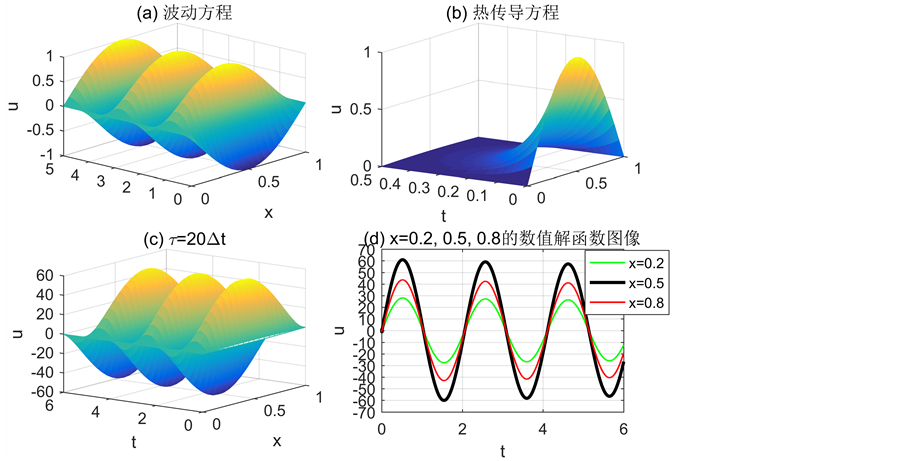Figure 3. When a, k = 1, string vibration equation (a), the heat conduction equation (b), memory dependent partial differential equations (c) and the numerical solution of memory-dependent partial differential equations in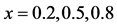(d)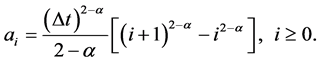5. 结论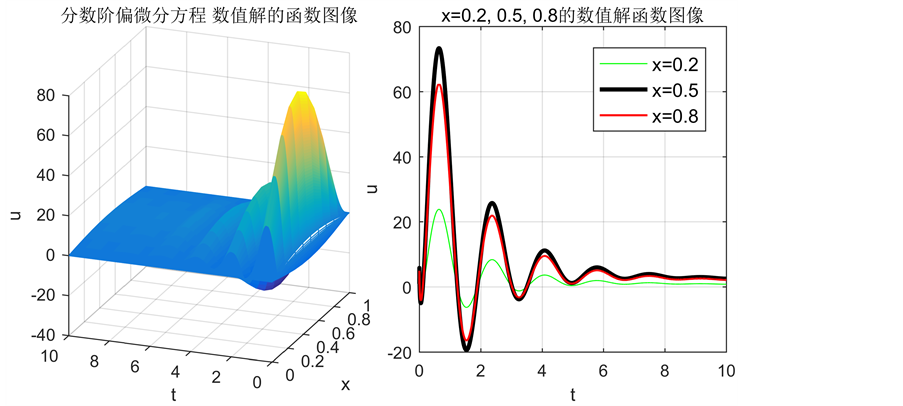Figure 4. Numerical solutions of FPDE composed of Caputo type differential operators when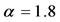andOn Numerical Solution of the Memory Dependent Partial Differential Equations[J]. 应用数学进展, 2017, 06(04): 637-643. http://dx.doi.org/10.12677/AAM.2017.64074

1. 1. Wang, J.L. and Li, H.F. (2011) Surpassing the Fractional Derivative: Concept of the Memory-Dependent Derivative. Computers and Mathematics with Applications, 62, 1562-1567. https://doi.org/10.1016/j.camwa.2011.04.028

2. 2. Ezzat, M.A., El-Karamany, A.S. and El-Bary, A.A. (2016) Generalized Thermos-Viscoelasticity with Memory-Dependent Derivatives Involving Two Tempera-tures. Mechanics of Advanced Materials and Structures, 23, 545-553. https://doi.org/10.1080/15376494.2015.1007189

3. 3. Ezzat, M.A. and El-Bary, A.A. (2016) Thermoelectric MHD with Memory-Dependent Derivative Heat Transfer. International Communications in Heat and Mass Transfer, 75, 270-281. https://doi.org/10.1016/j.icheatmasstransfer.2016.04.026

4. 4. Ezzat, M.A. and El-Bary. A.A. (2015) Memory-Dependent De-rivatives Theory of Thermo-Viscoelasticity Involving Two-Temperature. Journal of Mechanical Science and Technology, 29, 4273-4279. https://doi.org/10.1007/s12206-015-0924-1

5. 5. Li, H.F. and Wang, J.L. (2012) Molding the Dynamic System with Memo-ry-Dependent Derivative. 24th Chinese Control and Decision Conference (CCDC), Taiyuan, 23-25 May 2012, 1032-1036. https://doi.org/10.1109/CCDC.2012.6244162

6. 6. 王金良, 李宗军. 极点对称模态分解方法: 数据分析与科学探索的新途径[M]. 北京: 高等教育出版社, 2015.

7. 7. Wang, J.L. and Li, H.F. (2006) The Weighted Periodic Function and its Properties. Dynamics of Continuous Discrete and Impulsive Systems (Series A-Mathematical Analysis), 13, 1179-1183.

8. 8. 胡秀玲. 几类时间分数阶偏微分方程的有限差分方法研究[D]: [博士学位论文]. 南京: 南京航空航天大学, 2012.

9. 9. Sun, Z.Z. and Wu, X. (2006) A Fully Discrete Difference Scheme for a Diffusion-Wave System. Applied Numerical Mathematics, 56, 193-209. https://doi.org/10.1016/j.apnum.2005.03.003